## Thursday, September 21, 2017

### Simulating trees with specific values of Pybus & Harvey's γ

A colleague recently asked me the following question titled:

“Simulating trees with different pybus & Harvey's γ.”

“This may be a question with a very obvious answer, but is there a way of simulating Yule trees so they are either early burst or late burst (i.e. can you create simulated trees with a high or low γ)? Trying to satisfy a reviewerâ€™s request to test our taxonomy paper against null models.”

Well, we can't precisely simulate constant-rate pure-birth trees that have a particular γ value; however, one thing we could do is transform the edge lengths of the tree so that has a particular, predictable value of γ. In fact, it turns out to be the case - at least so far as I can tell (though I don't have a formal proof of this) that for any tree & particular value of γ, there is a corresponding “EB” (early-burst or ACDC, see Blomberg et al. 2003) edge-length transformation that will produce that very-same γ. It's just a matter of finding it!

Turns out - that's pretty easy. We can use numerical optimization, and since we only need to optimize in one-dimension, as the EB transformation only has one paramter, r, optimization is both fast & robust.

For instance:

``````library(phytools)
## simulate a tree
tree<-pbtree(n=100)
ltt(tree,show.tree=TRUE,log.lineages=F,log="y")
``````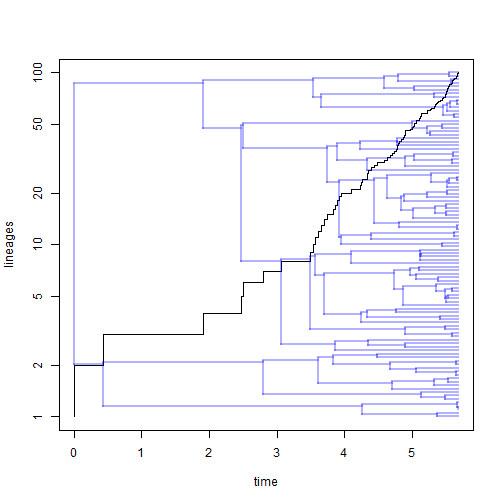``````## Object of class "ltt" containing:
##
## (1) A phylogenetic tree with 100 tips and 99 internal nodes.
##
## (2) Vectors containing the number of lineages (ltt) and branching times (times) on the tree.
##
## (3) A value for Pybus & Harvey's "gamma" statistic of 2.0956, p-value = 0.0361.
``````
``````## transform to gamma=0
ebTree<-phytools:::ebTree
gamma<-function(r,tree,g)
(g-ltt(ebTree(tree,r),plot=FALSE)\$gamma)^2
fit<-optimize(gamma,c(-10,10),tree=tree,g=0,tol=1e-12)
ltt(ebTree(tree,fit\$minimum),show.tree=TRUE,log.lineages=F,log="y",
lwd=2)
``````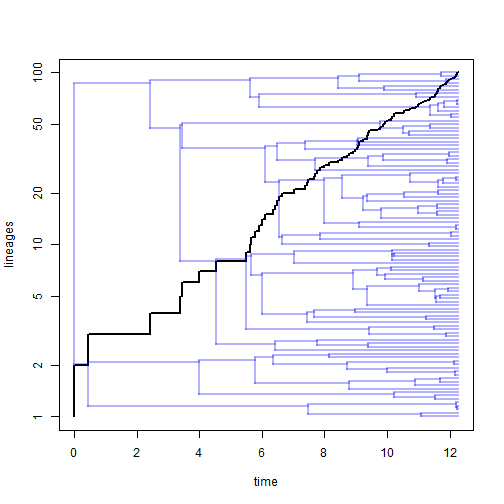``````## Object of class "ltt" containing:
##
## (1) A phylogenetic tree with 100 tips and 99 internal nodes.
##
## (2) Vectors containing the number of lineages (ltt) and branching times (times) on the tree.
##
## (3) A value for Pybus & Harvey's "gamma" statistic of 0, p-value = 1.
``````
``````## or how about gamma=10
fit<-optimize(gamma,c(-10,10),tree=tree,g=10,tol=1e-12)
ltt(ebTree(tree,fit\$minimum),show.tree=TRUE,log.lineages=F,log="y",
lwd=2)
``````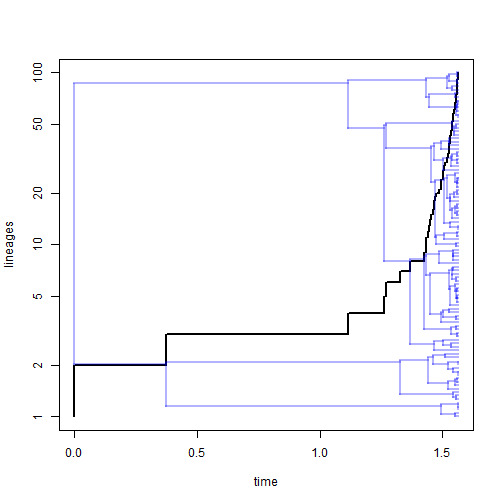``````## Object of class "ltt" containing:
##
## (1) A phylogenetic tree with 100 tips and 99 internal nodes.
##
## (2) Vectors containing the number of lineages (ltt) and branching times (times) on the tree.
##
## (3) A value for Pybus & Harvey's "gamma" statistic of 10, p-value = 0.
``````
``````## or gamma=-10
fit<-optimize(gamma,c(-10,10),tree=tree,g=-10,tol=1e-12)
ltt(ebTree(tree,fit\$minimum),show.tree=TRUE,log.lineages=F,log="y",
lwd=2)
``````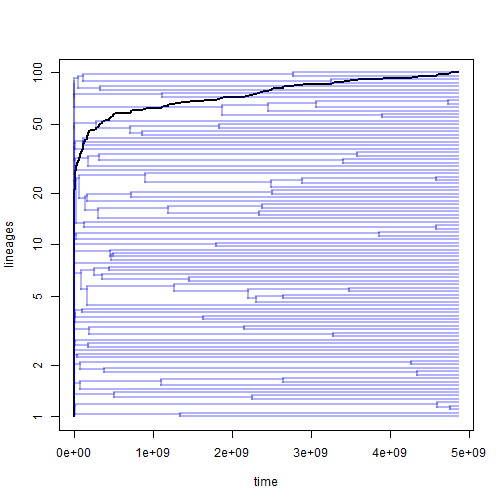``````## Object of class "ltt" containing:
##
## (1) A phylogenetic tree with 100 tips and 99 internal nodes.
##
## (2) Vectors containing the number of lineages (ltt) and branching times (times) on the tree.
##
## (3) A value for Pybus & Harvey's "gamma" statistic of -10, p-value = 0.
``````

Note that the `ebTree` transformation, though it does produce the correct γ, has the side-effect of changing the total depth of the tree. This needn't be the case. For instance, we can just do the following:

``````## custom EB function to hold total length constant
EB<-function(tree,r){
d<-max(nodeHeights(tree))
tree<-ebTree(tree,r)
tree\$edge.length<-tree\$edge.length/max(nodeHeights(tree))*d
tree
}
gamma<-function(r,tree,g)
(g-ltt(EB(tree,r),plot=FALSE)\$gamma)^2
## try with gamma=-4
fit<-optimize(gamma,c(-10,10),tree=tree,g=-4,tol=1e-12)
ltt(EB(tree,fit\$minimum),show.tree=TRUE,log.lineages=F,log="y",
lwd=2)
``````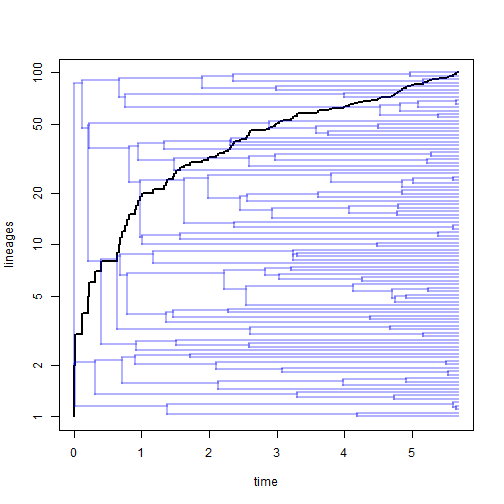``````## Object of class "ltt" containing:
##
## (1) A phylogenetic tree with 100 tips and 99 internal nodes.
##
## (2) Vectors containing the number of lineages (ltt) and branching times (times) on the tree.
##
## (3) A value for Pybus & Harvey's "gamma" statistic of -4, p-value = 1e-04.
``````

OK, now let's generate 100 trees with total length of 1.0 and γ=-1:

``````trees<-pbtree(n=100,scale=1,nsim=100)
foo<-function(tree,g)
optimize(gamma,c(-10,10),tree=tree,g=g,tol=1e-12)\$minimum
r<-sapply(trees,foo,g=-1)
ttrees<-mapply(EB,tree=trees,r=r,SIMPLIFY=FALSE)
class(ttrees)<-"multiPhylo"
g<-sapply(ttrees,function(x) ltt(x,plot=FALSE)\$gamma)
g
``````
``````##    -1 -1 -1 -1 -1 -1 -1 -1 -1 -1 -1 -1 -1 -1 -1 -1 -1 -1 -1 -1 -1 -1 -1
##   -1 -1 -1 -1 -1 -1 -1 -1 -1 -1 -1 -1 -1 -1 -1 -1 -1 -1 -1 -1 -1 -1 -1
##   -1 -1 -1 -1 -1 -1 -1 -1 -1 -1 -1 -1 -1 -1 -1 -1 -1 -1 -1 -1 -1 -1 -1
##   -1 -1 -1 -1 -1 -1 -1 -1 -1 -1 -1 -1 -1 -1 -1 -1 -1 -1 -1 -1 -1 -1 -1
##   -1 -1 -1 -1 -1 -1 -1 -1
``````
``````ltt(ttrees,col=make.transparent("blue",0.25))
``````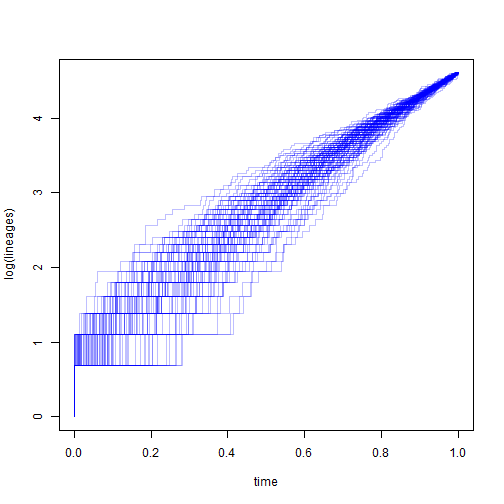``````## 100 objects of class "ltt" in a list
``````

What's neat is that for our single fixed value of γ = -1.0, the LTT curves vary quite a lot!

[Note. In the original post I called `ltt(trees,...)` rather than `ltt(ttrees,...)` - that is, `ltt` on the original, rather than transformed trees. Shown above this is fixed, but the LTT plots still vary quite a bit.]

That's it. Hopefully of some help.